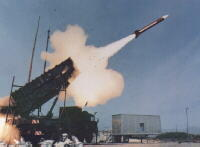# Calculator errors: When close isn’t close enough (Part 2)

In the previous post, I gave a simple classroom demonstration to illustrate that some calculators only approximate an infinite decimal expansion with a terminating decimal expansion, and hence truncation errors can propagate. This example addresses the common student question, “What’s the big deal if I round off to a few decimal places?”(For what it’s worth, I’m aware that some current high-end calculators are miniature computer algebra systems and can formally handle an answer of$\displaystyle \frac{1}{3}$ instead of its decimal expansion.)

Students may complain that the above exercise is artificial and unlikely to occur in real life. I would suggest following up with a real-world, non-artificial, and tragic example of an accident that happened in large part due to truncation error. This incident occurred during the first Gulf War in 1991 (perhaps ancient history to today’s students). I’m going to quote directly from the website http://www.ima.umn.edu/~arnold/disasters/patriot.html, published by Dr. Douglas Arnold at the University of Minnesota. Perhaps students don’t need to master the details of this explanation (a binary expansion as opposed to a decimal expansion might be a little abstract), but I think that this example illustrates truncation error vividly.

On February 25, 1991, during the Gulf War, an American Patriot Missile battery in Dharan, Saudi Arabia, failed to track and intercept an incoming Iraqi Scud missile. The Scud struck an American Army barracks, killing 28 soldiers and injuring around 100 other people.A report of the General Accounting office, GAO/IMTEC-92-26, entitled Patriot Missile Defense: Software Problem Led to System Failure at Dhahran, Saudi Arabia reported on the cause of the failure.

It turns out that the cause was an inaccurate calculation of the time since boot due to computer arithmetic errors. Specifically, the time in tenths of second as measured by the system’s internal clock was multiplied by$1/10$ to produce the time in seconds. This calculation was performed using a 24 bit fixed point register. In particular, the value$1/10$, which has a non-terminating binary expansion, was chopped at 24 bits after the radix point. The small chopping error, when multiplied by the large number giving the time in tenths of a second, led to a significant error.

Indeed, the Patriot battery had been up around 100 hours, and an easy calculation shows that the resulting time error due to the magnified chopping error was about$0.34$ seconds.

The number$1/10$ equals$\displaystyle \frac{1}{2^4} + \frac{1}{2^5} +\frac{1}{2^8} + \frac{1}{2^9} + \frac{1}{2^{12}} + \frac{1}{2^{13}} + \dots$

In other words, the binary expansion of$1/10$ is$0.0001100110011001100110011001100....$

Now the 24 bit register in the Patriot stored instead$0.00011001100110011001100$

introducing an error of$0.0000000000000000000000011001100...$ binary,

or about$0.000000095$ decimal. Multiplying by the number of tenths of a second in$100$ hours gives$0.000000095 \times 100 \times 60 \times 60 \times 10=0.34$.

A Scud travels at about$1,676$ meters per second, and so travels more than half a kilometer in this time. This was far enough that the incoming Scud was outside the “range gate” that the Patriot tracked.

Ironically, the fact that the bad time calculation had been improved in some parts of the code, but not all, contributed to the problem, since it meant that the inaccuracies did not cancel.

The following paragraph is excerpted from the GAO report.

The range gate’s prediction of where the Scud will next appear is a function of the Scud’s known velocity and the time of the last radar detection. Velocity is a real number that can be expressed as a whole number and a decimal (e.g., 3750.2563…miles per hour). Time is kept continuously by the system’s internal clock in tenths of seconds but is expressed as an integer or whole number (e.g., 32, 33, 34…). The longer the system has been running, the larger the number representing time. To predict where the Scud will next appear, both time and velocity must be expressed as real numbers. Because of the way the Patriot computer performs its calculations and the fact that its registers are only 24 bits long, the conversion of time from an integer to a real number cannot be any more precise than 24 bits. This conversion results in a loss of precision causing a less accurate time calculation. The effect of this inaccuracy on the range gate’s calculation is directly proportional to the target’s velocity and the length of the the system has been running. Consequently, performing the conversion after the Patriot has been running continuously for extended periods causes the range gate to shift away from the center of the target, making it less likely that the target, in this case a Scud, will be successfully intercepted.A quick note of clarification. To verify the binary expansion of$1/10$, we use the formula for an infinite geometric series.$S = \displaystyle \left(\frac{1}{2^4} + \frac{1}{2^5}\right) +\left(\frac{1}{2^8} + \frac{1}{2^9}\right) + \left(\frac{1}{2^{12}} + \frac{1}{2^{13}}\right) + \dots$$S = \displaystyle \frac{3}{2^5} + \frac{3}{2^9} + \frac{3}{2^{13}} + \dots$$S = \displaystyle \frac\frac{3}{2^5}}{\quad \displaystyle 1 - \frac{1}{2^4} \quad$$S = \displaystyle \frac\frac{3}{32}}{\quad \displaystyle \frac{15}{16} \quad$$S = \displaystyle \frac{3}{32} \times \frac{16}{15}$$S = \displaystyle \frac{1}{10}$

OK, that verifies the answer. Still, a curious student may wonder how one earth one could directly convert$1/10$ into binary without knowing the above series ahead of time. I will address this question in a future post.

Leave a comment

### 3 Comments

This site uses Akismet to reduce spam. Learn how your comment data is processed.